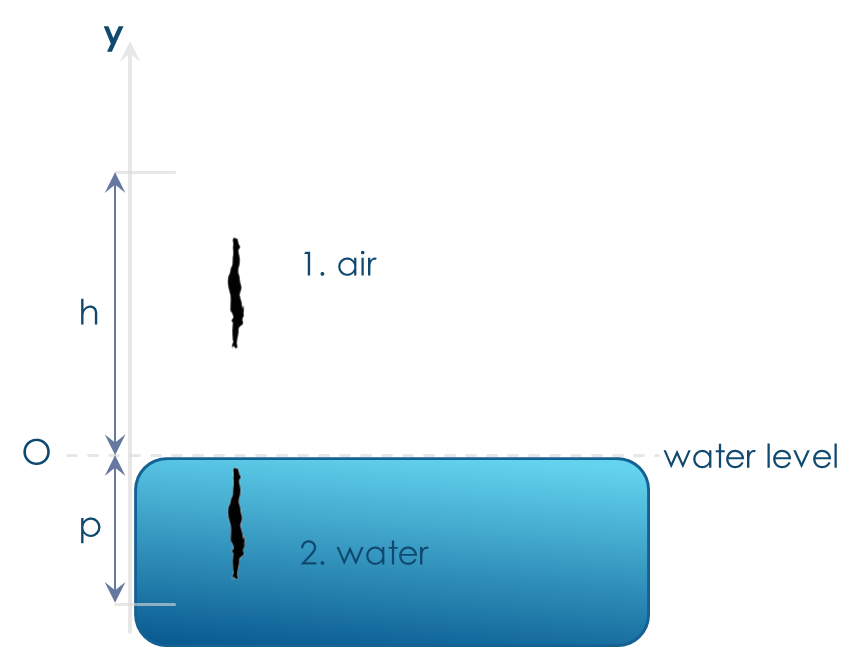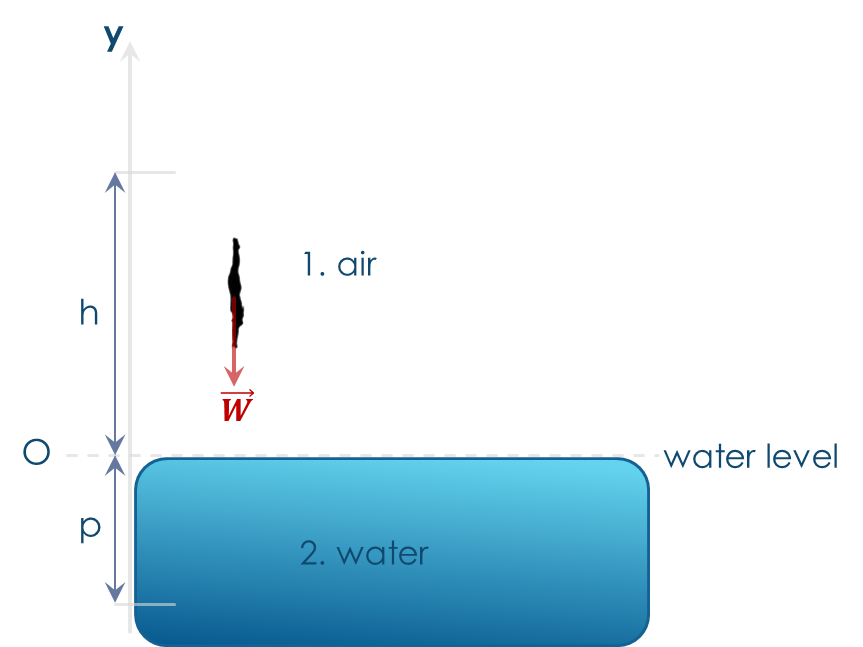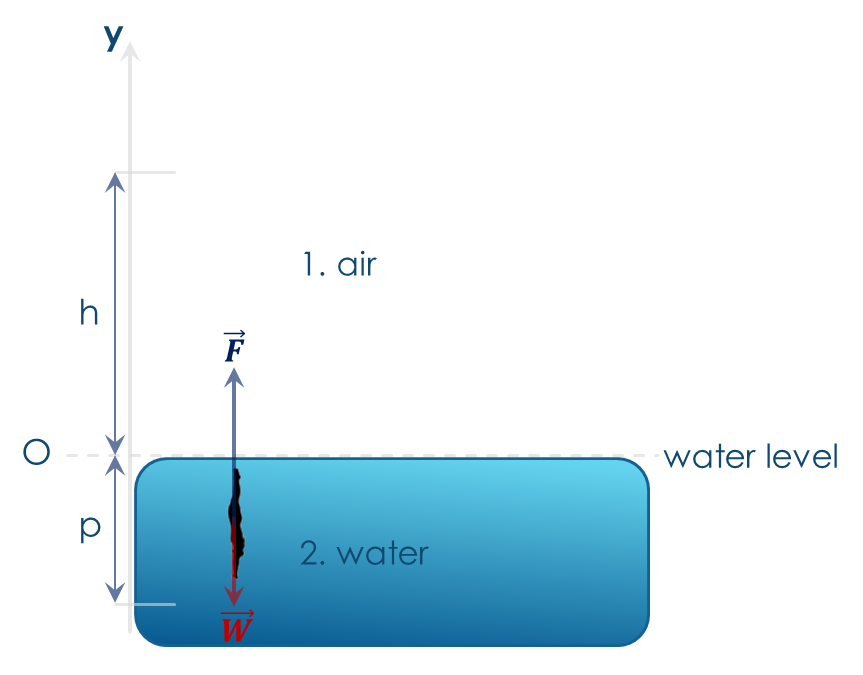# Problem statement

A 60kg man dives in a pool from a 10m trampoline.

Assuming he starts being still and neglecting his upwards jump, find his velocity when he reaches the water.

Find the average force the water exerts on him, if he​​ stops at 5m under water.Fig.1

In the picture, a​​ reference system​​ has been chosen and the​​ origin​​ (O) has been fixed at the water level. Since the motion happens along one direction only (vertical direction), we need to use only one vertical axis, the traditional ‘y’ axis, on which the upward arrow has been chosen as the positive orientation of the axis: this means that all vectors directed upwards will be positive while all vectors directed downwards will be negative.

Let’s assume, since there’s no mention in the problem statement, that there’s​​ no air friction. This hypothesis, used in all basic physics courses, will significantly simplify the problem solution.

Motion can be divided in 2 parts:

• Motion in the air

• Motion in the water

So, we’ll divide the problem solution in 2 parts accordingly.

## PART 1: MOTION IN THE AIR

First of all, let’s try to understand​​ which forces act on the diving man. Since we assumed no friction in the air,​​ the only force acting on the man is the gravity, that is, the man’s weight (fig. 2).

Weight is approximately constant if the man is close enough to the Earth surface, so Newton’s law assures the acceleration will be constant: g

W=mg=-mgy^

Therefore, the man will experience a​​ uniformly accelerated motion, with an acceleration

g=-9.81ms2y^

Since this​​ motion is in one dimension (y) – for simplicity of notation – we’ll avoid the vector notation (y^) unless necessary.Fig.2

The time law is:

y=y0+v0t-12gt2

Where:

y0=h=10m,v0=0,g=9.81ms2

That is:

y=h-12gt2

Setting​​ y=0​​ (water level), we can get the time at which the man will touch the water:

0=h-12gt2

 t= 2hg (1)

In a constant acceleration motion, the speed-acceleration relationship is:

v=v0+at

Where​​ v0=0.​​ So, from (1):

v=at=gt= g2hg= 2hg2g=

=​​ 2gh=14ms

## PART 2: MOTION IN THE WATER

The average force exerted by the water on the man is - by definition - a​​ constant force​​ having the same effect of the​​ real water force, that is stopping the man at 5m under the water.

So, we’ll find the constant force which stops the man at 5m under waterFig. 3

Again, let’s try to understand​​ which forces act on the man in the water. He undergoes 2 forces (fig. 3): his​​ weight​​ W​​ and the water constant force​​ F, which can be written (in vectorial notation) as follows

W=mg=-mgy^

F=Fy^

The Newton’s dynamics law can be written as:

W+F=ma

and, since both the weight and the water force are constant, the man acceleration in the water will be constant too.

So we have a​​ constant deceleration motion, with starting velocity

v0=-14msy^

Again, motion is in one dimension (y) so, we’ll avoid the vector notation (y^) unless necessary.

So, space and velocity laws are:

s=s0+v0t+12at2v= v0+at

Where​​ s0=0,​​ v0=-14ms​​ (directed downwards), acceleration a is​​ unknown but it’s directed upwards since the man stops.

We need to solve previous equations for​​ v=0​​ (the man stops) and​​ s=p=-5m.​​ So, we need to solve

-5=-14t+12at20=-14+at

From the second equation we have​​ at=14. Substituting in the first, we get

-5=-14t+142t

t=0.71s

Substituting​​ t=0.71s in the second equation, we​​ get

a=19.61ms2,

directed upwards.

Going back to the Newton’s dynamics law, we have:

W+F=ma

mg+F= ma

F= ma -mg

F= ma -mg=ma -g=

=60kg19.6ms2--9.81ms2y^=

=60kg×29.41ms2y^=1765Ny^